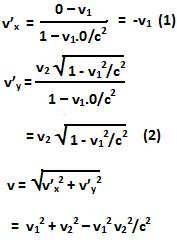## Saturday, November 21, 2009

### Irodov Problem 1.361

In this problem we can use the solution we found in Problem 1.358, namelyLet us find the velocity of the particle moving along the y-axis relative to the particle moving on the x-axis. For this, in our above equations we have to substitute, V= v1 , vx=0, vy=v2. Thus, we have, the relative velocity is given by,